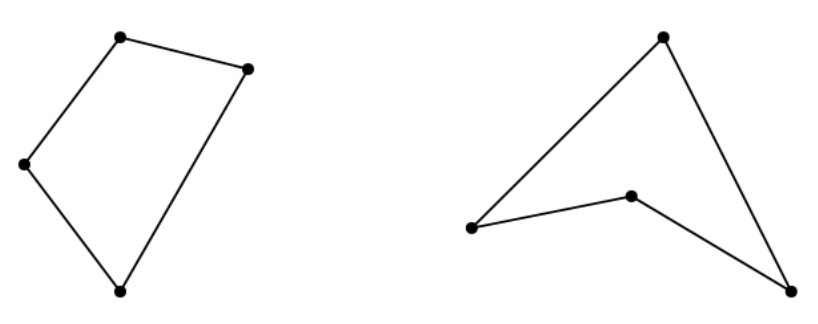시간 제한메모리 제한제출정답맞힌 사람정답 비율
1.7 초 512 MB222835531.792%

## 문제

A quadrilateral is a polygon with exactly four distinct corners and four distinct sides, without any crossing between its sides. A quadrilateral is called convex if all the inner angles at its corners are less than 180 degrees, or called non-convex, otherwise. See the illustration below for a convex quadrilateral (left) and a non-convex quadrilateral (right).In a test problem, you are given a set P of n points in the plane, no three of which are collinear, and asked to find as many quadrilaterals as possible by connecting four points from P, while each point in P can be used limitlessly many times and those quadrilaterals you find may freely overlap each other. You will earn different credits for each quadrilateral you find, depending on its shape and area. In principle, you earn more credits for convex quadrilaterals and for quadrilaterals with minimum area.

More precisely, the rules for credits are as follows, where a denotes the minimum over the areas of all possible quadrilaterals formed by connecting four points in P:

• For each distinct convex quadrilateral with area exactly a, you earn 4 credits.
• For each distinct non-convex quadrilateral with area exactly a, you earn 3 credits.
• For each distinct convex quadrilateral with area strictly larger than a, you earn 2 credits.
• For each distinct non-convex quadrilateral with area strictly larger than a, you earn 1 credit.

Note that two quadrilaterals are distinct unless the corners and sides of one are exactly the same to the other’s, and that there may be two or more quadrilaterals of the minimum area a.

You of course want to find all possible quadrilaterals and earn the maximum possible total credits. Given a set P of n points in the plane, write a program that outputs the maximum possible total credits you can earn when you find all possible quadrilaterals from the set P.

## 입력

Your program is to read from standard input. The input starts with a line containing an integer n (4 ≤ n ≤ 1,000), where n denotes the number of points in the set P. In the following n lines, each line consists of two integers, ranging −109 to 109, representing the coordinates of a point in P. There are no three points in P that are collinear.

## 출력

Your program is to write to standard output. Print exactly one line consisting of a single integer that represents the maximum possible total credits you can earn from the set P.

## 예제 입력 1

4
0 0
1 0
0 1
1 1


## 예제 출력 1

4


## 예제 입력 2

4
0 0
10 0
5 10
5 8


## 예제 출력 2

5


## 예제 입력 3

4
0 0
10 0
5 10
5 3


## 예제 출력 3

7


## 예제 입력 4

5
0 0
0 5
5 0
5 5
4 2


## 예제 출력 4

14